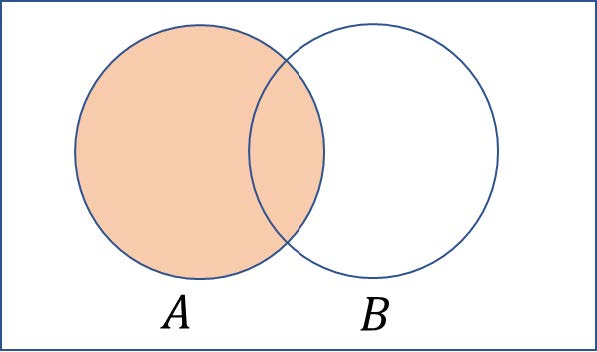# P(A) = p, P(B) = 2p, P(Exactly one out of A and B occurs) = 5/9. What will be the maximum value of p?Solution:

Given: P(A) = p and P(B) = 2p

$$P(\bar{A} \cap B) + P(\bar{B} \cap A) = \frac{5}{9}$$

P(A)-P(A∩B)+P(B)-P(A∩B) = 5/9

3p-2P(A∩B) = 5/9

0 ≤ P(A∩B) ≤ p

For maximum value: 3p – 2p = 5/9

So, p = 5/9(0)(0)